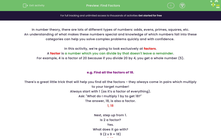# Find Factors

In this worksheet, students will practise finding factors of numbers with up to two digits to aid application and identification of these special numbers in problems.Key stage:  KS 4

Year:  GCSE

GCSE Subjects:   Maths

GCSE Boards:   Pearson Edexcel, OCR, Eduqas, AQA,

Curriculum topic:   Number, Number Operations and Integers

Curriculum subtopic:   Structure and Calculation Whole Number Theory

Popular topics:   Numbers worksheets, Prime Numbers worksheets, Square Numbers worksheets,

Difficulty level:#### Worksheet Overview

In number theory, there are lots of different types of numbers: odds, evens, primes, squares, etc.

An understanding of what makes these numbers special and knowledge of which numbers fall into these categories can help you solve complex problems quickly and with confidence.

In this activity, we're going to look exclusively at factors.

A factor is a number which you can divide by that doesn't leave a remainder.

For example, 4 is a factor of 20 because if you divide 20 by 4, you get a whole number (5).

e.g. Find all the factors of 18.

There's a great little trick that will help you find all the factors - they always come in pairs which multiply to your target number!

Ask: "What do I multiply 1 by to get 18?"

The answer, 18, is also a factor.

1, 18

Next, step up from 1.

Is 2 a factor?

Yes.

What does it go with?

9 (2 x 9 = 18)

1, 18

2, 9

Step it up again.

Is 3 a factor?

Yes.

What does it go with?

6 (3 x 6 = 18)

1, 18

2, 9

3, 6

Is 4 a factor?

No.

Is 5 a factor?

No

So why can I stop now?

The next number I would look at is 6 which I already know is a factor.

My numbers on the left go up, the numbers on the right come down and they eventually meet in the middle.

Job done!

So the factors of 18 are: 1, 2, 3, 6, 9, 18.

e.g. Find all the factors of 36.

If we follow the same idea as before, starting at 1 and working our way up, we should get the following factor pairs.

1 x 36

2 x 18

3 x 12

4 x 9

6 x 6

So the factors of 36 are: 1, 2, 3, 4, 6, 9, 12, 18 and 36.

Be careful not to write 6 twice, as it can only be a factor once!

In this activity, you will apply the process described above to find factors of numbers with up to two digits.

### What is EdPlace?

We're your National Curriculum aligned online education content provider helping each child succeed in English, maths and science from year 1 to GCSE. With an EdPlace account you’ll be able to track and measure progress, helping each child achieve their best. We build confidence and attainment by personalising each child’s learning at a level that suits them.

Get started